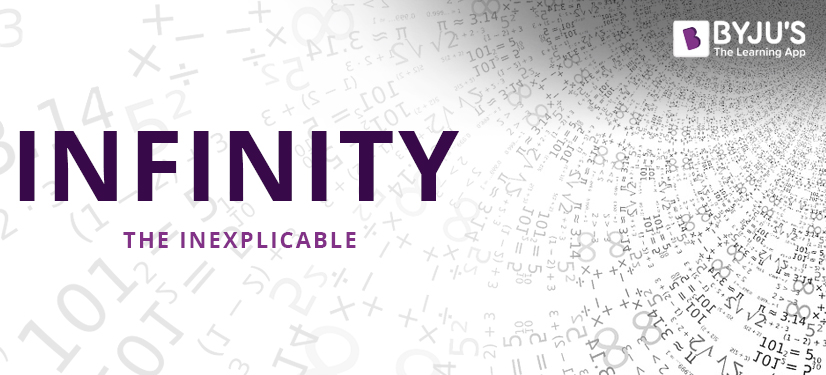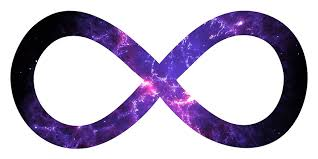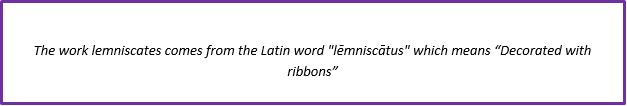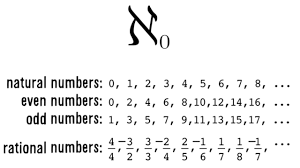# Infinity- The InexplicableTime and again, the term “infinite” is known to have blown some of the major minds in the disciplines of philosophy, metaphysics and mathematics since its inception. Infinity is the idea of something that has no end. In layman’s language, it can be called unbounded, indefinite, undefined, inconceivable, formless, endless, eternity – the only limit is one’s imagination!

“Infinity is a floorless room without walls or ceiling.”

If asked, what could be the biggest possible number that you can name?

Probably a quintillion, a decillion or a centillion (10600)? Or even a Googol, a Googleplex or a Googolplexian  (Look at that figure!)

But at the end of the day, all these numbers are finite. None of them are even close to infinity, because one could eventually ‘get there’. And infinity is not definite- A wonder.

The speculation of infinity is often said to have begun with the ancient Greeks, but they were reluctant to accept the concept of actual infinite in quantity. Their imagination extended only up to potential infinity. It was Zeno of Elea, in 450 BC, who brought to the fore his paradoxes involving infinity. Since then, significant researches and severe brainstorming has been done by the mathematicians, and more subtle paradoxes were conceived.English mathematician, John Wallis is credited with the introduction of the infinity symbol in 1655. The symbol ∞, which is the lazy eight curve, is called the lemniscates.Although the concept of infinity itself is abstract, can one set of infinity be more infinite than another set of infinity? Confused?

Though infinity is boundless, yet it can be in different sizes. For example:

The positives numbers (greater than zero) and the negative numbers (smaller than zero) can be considered as two sets of infinite sets of equal size. But what if we combine both of these sets together? We’ll get an infinite set twice as large the individual sets of both positive and negative numbers.

Another example would be the infinite set of even numbers. It will represent an infinity set half the size of all the whole numbers.The assumption that something truly infinite exists in nature can be categorized into “infinitely big” and “infinitely small.” Like, space can have infinite volume, time can continue forever or there can be infinitely many physical objects. They are termed as the infinitely big ones. Similarly, infinitely small can be defined by the idea that even a liter of space contains an infinite number of points. It has been quite a struggle to understand the pre-existing concepts and merge them with the modern ideas to use the notion of infinity in a coherent manner.

However, despite being a subject of research for over 2000 years, the existence of infinity still remains questionable. Does it really exist, or is it just a fabric of our imaginations? Will the tantalizing mystery of infinite enigma ever be resolved to the satisfaction of logicians and mathematicians?

Infinity is undoubtedly one of the strangest, richest and most dangerous notions mankind has invented. Here is how a fourth-grade student explained infinity: “Infinity is a number that is impossible to count. It is as many atoms as there are on planet earth, or in the Solar System. Infinity is how many raindrops are there in a downpour. It is how many pages of homework you get in school. It is how far it is to reach the end of a circle. Infinity is how far it is to the farthest star. Infinity is how long it is, till there is peace in all the world and universe!”

Sankalp

October 5, 2018

Why didn’t students comment on this topic? However if someone is seeing this please reply.
I am not totally satisfied with statement ‘ The infinite set of even numbers will represent an infinity set half the size of all the whole numbers.’
It may seem true but it is not. If we compare each no. from both set they are 1 to 1 i.e.
From first no. : W-0, E-0
Second no. : W-1, E-2
Third no. : W-2,E4. And so on… Since there are many numbers there is a no. for each whole number.

Shivi

July 22, 2018

Interesting!??

Testimonials

### Book Your Free Class Now